Question 1: Solve the following systems of linear inequations graphically:

i)$2x+3y \leq 6 , \hspace{0.3cm} 3x+2y \leq 6, \hspace{0.3cm}x \geq 0, y \geq 0$

ii)$2x+3y \leq 6 , \hspace{0.3cm} x+4y \leq 4, \hspace{0.3cm}x \geq 0, y \geq 0$

iii)$x-y \leq 1 , \hspace{0.3cm} x+2y \leq 8, \hspace{0.3cm} 2x+y \geq 2, \hspace{0.3cm}x \geq 0, y \geq 0$

iv)$x+y \geq 1 , \hspace{0.3cm} 7x+9y \leq 63, \hspace{0.3cm} x \leq 6, \hspace{0.3cm} y \leq 5, \hspace{0.3cm}x \geq 0, y \geq 0$

v)$2x+3y \leq 35 , \hspace{0.3cm} y \geq 3, \hspace{0.3cm} \hspace{0.3cm}x \geq 2, x \geq 0, y \geq 0$

i)$2x+3y \leq 6 , \hspace{0.3cm} 3x+2y \leq 6, \hspace{0.3cm}x \geq 0, y \geq 0$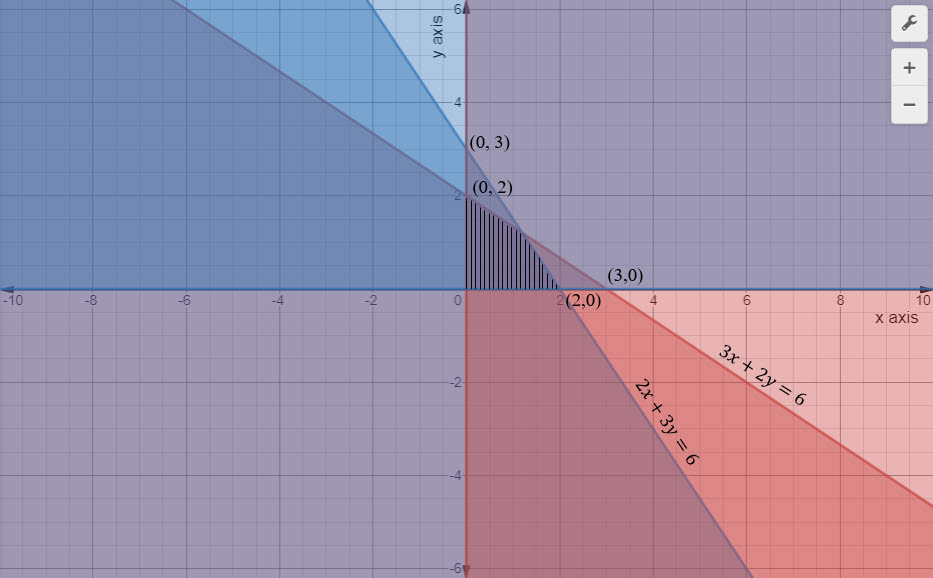ii)$2x+3y \leq 6 , \hspace{0.3cm} x+4y \leq 4, \hspace{0.3cm}x \geq 0, y \geq 0$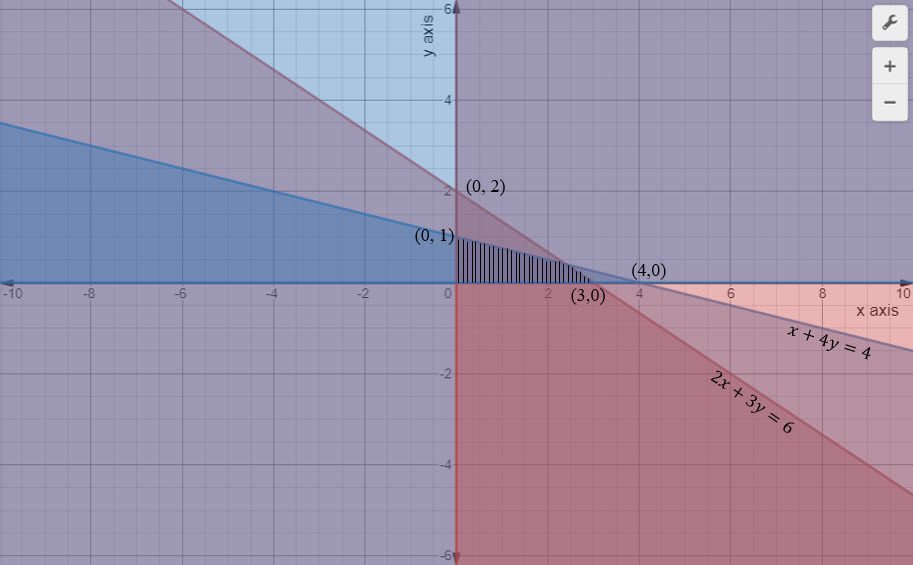iii)$x-y \leq 1 , \hspace{0.3cm} x+2y \leq 8, \hspace{0.3cm} 2x+y \geq 2, \hspace{0.3cm}x \geq 0, y \geq 0$iv)$x+y \geq 1 , \hspace{0.3cm} 7x+9y \leq 63, \hspace{0.3cm} x \leq 6, \hspace{0.3cm} y \leq 5, \hspace{0.3cm}x \geq 0, y \geq 0$v)$2x+3y \leq 35 , \hspace{0.3cm} y \geq 3, \hspace{0.3cm} \hspace{0.3cm}x \geq 2, x \geq 0, y \geq 0$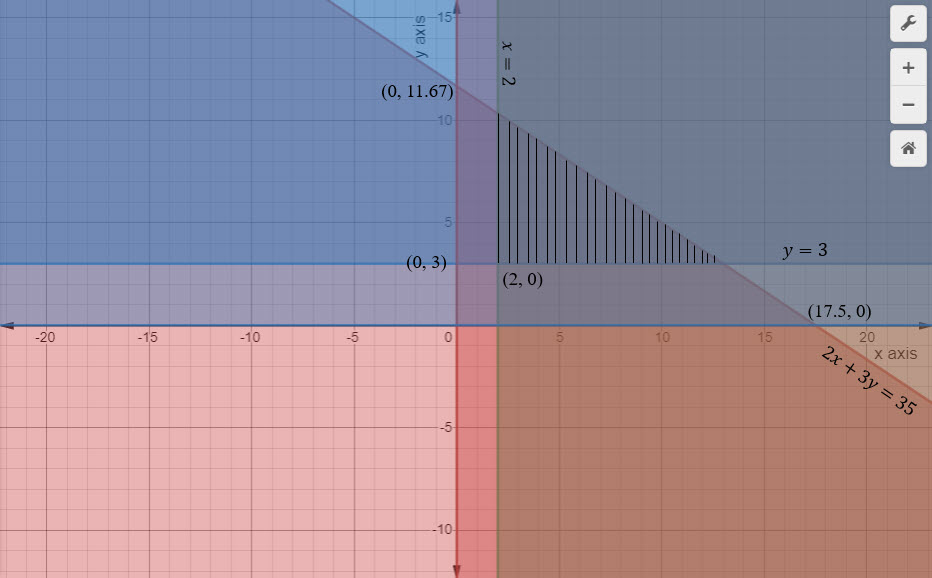$\\$

Question 2: Show that the solution set of the following linear inequations is empty set:

i)$x-2y \geq 0 , \hspace{0.3cm} 2x-y \leq -2, \hspace{0.3cm}x \geq 0, y \geq 0$

ii)$x+2y \leq 3 , \hspace{0.3cm} 3x+4y \geq 12, \hspace{0.3cm} y \geq 1, \hspace{0.3cm} x \geq 0, y \geq 0$

i)$x-2y \geq 0 , \hspace{0.3cm} 2x-y \leq -2, \hspace{0.3cm}x \geq 0, y \geq 0$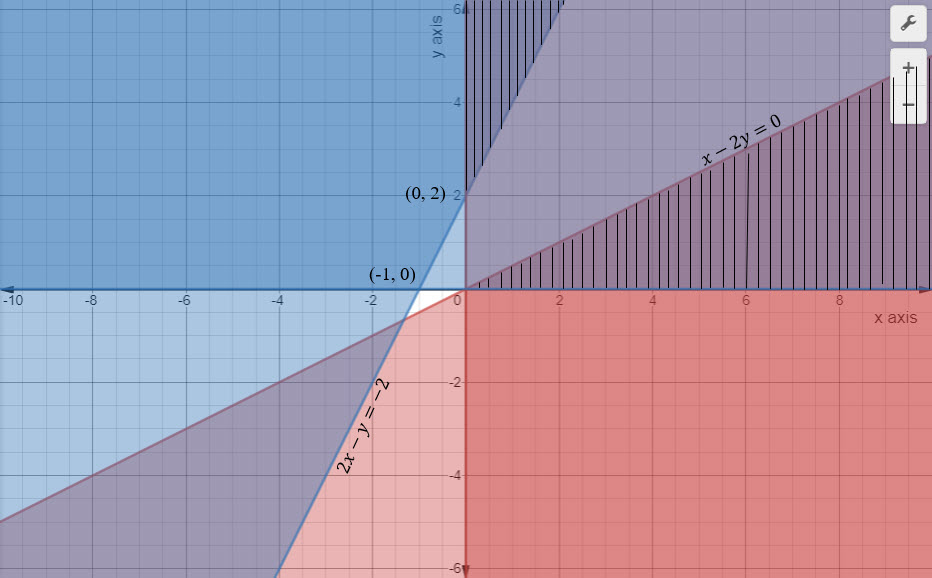There is no common areas between the inequations. Hence there is no solution or the solution is null set.

ii)$x+2y \leq 3 , \hspace{0.3cm} 3x+4y \geq 12, \hspace{0.3cm} y \geq 1, \hspace{0.3cm} x \geq 0, y \geq 0$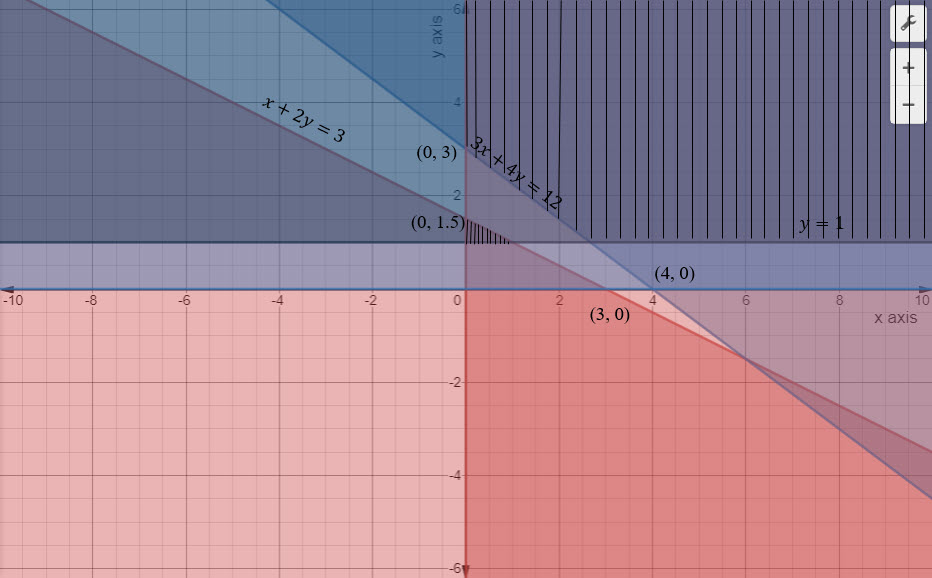There is no common areas between the inequations. Hence there is no solution or the solution is null set.$\\$

Question 3: Find the linear inequations for which the shaded area in the figure below is the solution set. Draw the diagram of the solution set of the linear in-equations.Answer:

The inequations are$4x+6y \leq 24, \ \ \ -3x+2y \leq 3 , \ \ \ x - 2y \leq 2, \ \ \ 2x+ 3y \geq 6$$\\$

Question 4: Find the linear inequations for which the solution set is the shaded region given in the figure below.Answer:

The inequations are$x+y \leq 4, \ \ \ y \leq 3 , \ \ \ x \leq 3, \ \ \ 6x+2y \geq 8 \ \ \ x \geq 0, \ \ \ y\geq 0$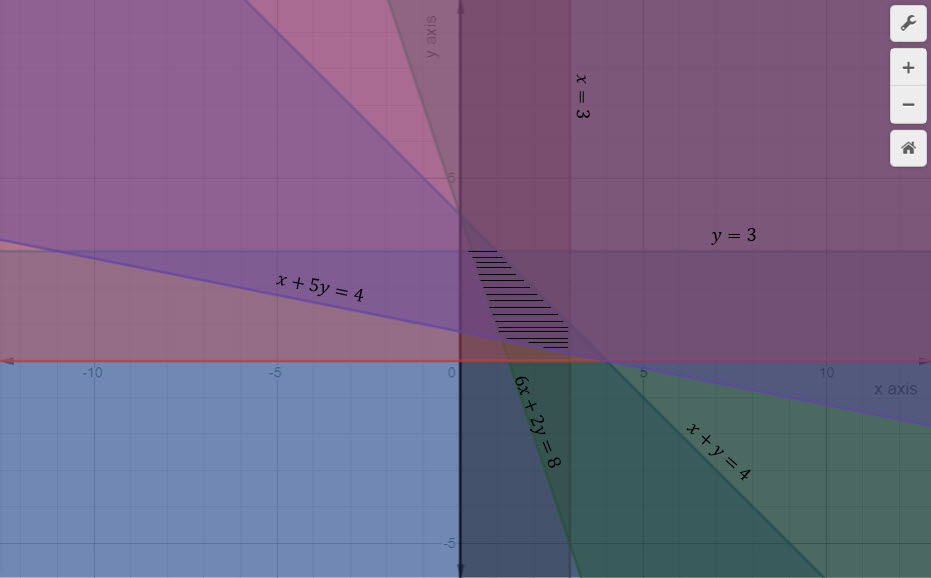$\\$

Question 5: Show that the solutions set of the following linear inequations is an unbound set:$x+y \geq 9 , \hspace{0.3cm} 3x+y \geq 12, \hspace{0.3cm}x \geq 0, y \geq 0$

Solutions set of the following linear inequations is an unbound set.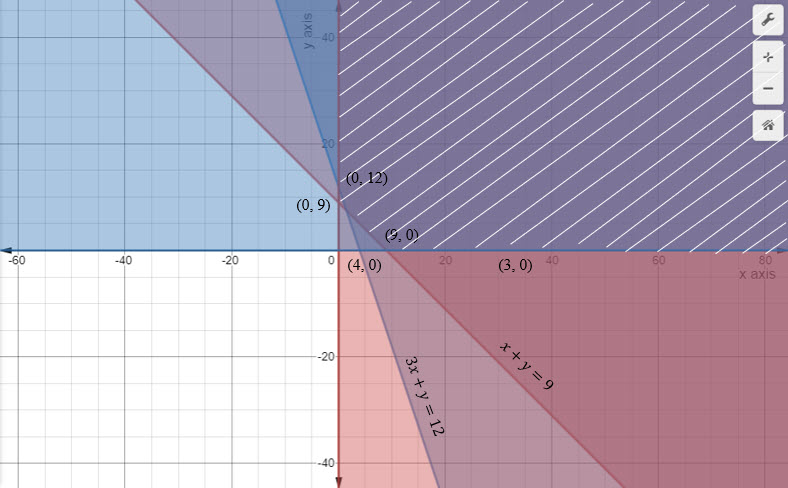$\\$

Question 6: Solve the following systems of inequations graphically:

i)$2x+y \geq 8, \hspace{0.3cm} x + 2y \geq 8, \hspace{0.3cm} x + y \leq 6$

ii)$12x+12y \leq 840, \hspace{0.3cm} 3x + 6y \leq 300, \hspace{0.3cm} 8x+4y \leq 480, \hspace{0.3cm} x \geq 0, y \geq 0$

iii)$x+2y \leq 40, \hspace{0.3cm} 3x + y \geq 30, \hspace{0.3cm} 4x+3y \geq 60, \hspace{0.3cm} x \geq 0, y \geq 0$

iv)$5x+y \geq 10, \hspace{0.3cm} 2x + 2y \geq 12, \hspace{0.3cm} x+4y \geq 12, \hspace{0.3cm} x \geq 0, y \geq 0$

i)$2x+y \geq 8, \hspace{0.3cm} x + 2y \geq 8, \hspace{0.3cm} x + y \leq 6$ii)$12x+12y \leq 840, \hspace{0.3cm} 3x + 6y \leq 300, \hspace{0.3cm} 8x+4y \leq 480, \hspace{0.3cm} x \geq 0, y \geq 0$iii)$x+2y \leq 40, \hspace{0.3cm} 3x + y \geq 30, \hspace{0.3cm} 4x+3y \geq 60, \hspace{0.3cm} x \geq 0, y \geq 0$iv)$5x+y \geq 10, \hspace{0.3cm} 2x + 2y \geq 12, \hspace{0.3cm} x+4y \geq 12, \hspace{0.3cm} x \geq 0, y \geq 0$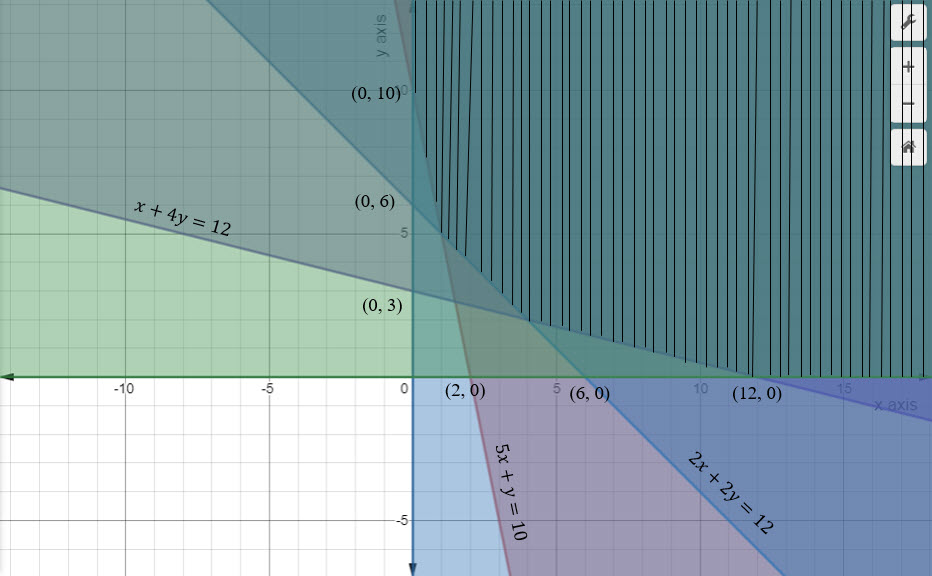$\\$

Question 7: Show that the following system of linear inequations have some solution:$x+2y \leq 3, \hspace{0.3cm} 3x + 4y \geq 12, \hspace{0.3cm} x \geq 0, y \geq 1$

Answer:There is no solution set for the given inequalities.$\\$

Question 8:  Show that the solution set of the following system of linear inequations is an unbounded region$2x+y \geq 8, \hspace{0.3cm} x + 2y \geq 10, \hspace{0.3cm} x \geq 0, y \geq 0$

We see that the solution set is the unbounded region.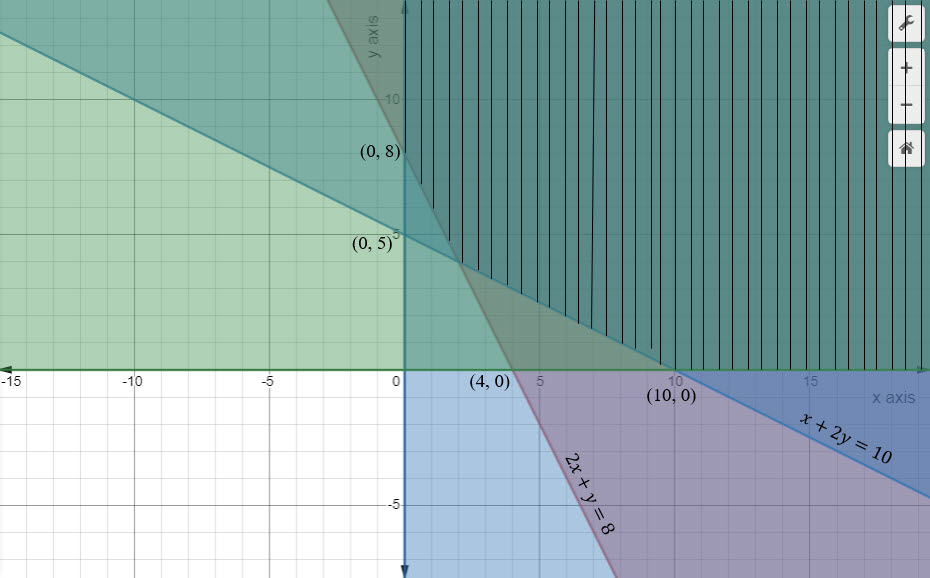$\\$